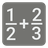Fractions Addition
Simple
English RU

Your task is to write a function which takes the tuple of tuples containing fractions as an argument and returns the sum of those fractions. The fractions will look like this: (x, y), where 'x' is the numerator, and 'y' is the denominator. For example, (2, 3) means 2/3. If the numerator is greater than the denominator (after the addition)...
fractions (((2, 3), (2, 3))) = "1 and 1/3", because the result will be - 4/3 (the numerator is greater than the denominator) and you can extract the integer part (1) and the remaining fraction (1/3). Make note that the conjunction 'and' is required if the result has both parts - the integer and the fraction.
If the result doesn’t contain the fraction part and has only the integer - you should return it as the 'int'-type, not 'str'. If it doesn’t contain the integer part - just return it like a string 'N/D' where N - is the numerator and D - is the denominator.

You should be an authorized user in order to see the full description and start solving this mission.